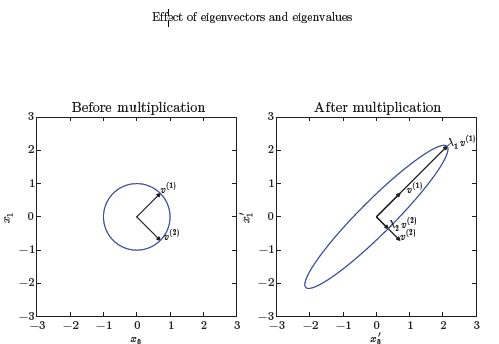# 深度学习/机器学习入门基础数学知识整理（一）：线性代数基础，矩阵，范数等

## 1.线性代数

1.1 标量，向量，矩阵和张量

• 标量（scalar）：一个标量就是一个单独的数。用斜体表示标量，如 sR $s\in R$.
• 向量（vector）：一个向量是一列数，我们用粗体的小写名称表示向量。比如
x，将向量x 写成方括号包含的纵柱：

x=x1x2xn

• 矩阵（matrix）：矩阵是二维数组，我们通常赋予矩阵粗体大写变量名称，比如
A​ 。如果一个矩阵高度是m​，宽度是n​，那么说 ARm×n $A \in R^{m×n}$ 。一个矩阵可以表示如下：

A=[x11x21x12x22]

• 张量（tensor）：某些情况下，我们会讨论不止维坐标的数组。如果一组数组中的元素分布在若干维坐标的规则网络中，就将其称为张量。用A​ 表示，如张量中坐标为(i,j,k)​的元素记作 Ai,j,k $A_{i,j,k}$

• 转置（transpose）：矩阵的转置是以对角线为轴的镜像，这条从左上角到右下角的对角线称为主对角线（main diagonal）。将矩阵A的转置表示为 AT $A^T$。定义如下： (A)i,j=Aj,i $({\bf A^\top})_{i,j}=\bf A_{j,i}$

A=x11x21x31x12x22x32A=[x11x21x21x22x31x32]

1.2 矩阵和向量相乘

• 矩阵乘法：矩阵运算中最重要的操作之一。两个矩阵A和B 的矩阵乘积(matrix product)是第三个矩阵C 。矩阵乘法中A 的列必须和 B的行数相同。即如果矩阵 A的形状是 m×n，矩阵B的形状是 n×p，那么矩阵 C的形状就是 m×p。即

C=A×B
具体的地，其中的乘法操作定义为

Ci,j=kAi,kBk,j
矩阵乘积服从分配律，结合律，但是矩阵乘法没有交换律，

A(B+C)=AB+ACA(BC)=(AB)CABBA

• 点积（dot product）两个相同维数的向量x和y的点积可看作是矩阵乘积 xTy $x^Ty$

• 矩阵乘积的转置 (向量也是一种特殊的矩阵)

(AB)=BAxy=(xy)=yx

1.3 单位矩阵和逆矩阵

A1A=AA1=InInA=A

1.4 范数

||x||p=(i|xi|p)1ppR,p1

f(x)=0x=0f(x+y)f(x)+f(y)αR,f(αx)=|α|f(x)

max范数（max norm）：这个范数表示向量中具有最大幅度得元素的绝对值，用 L $L_\infty$范数表示，形式为：

||x||=max|xi|

xy=||x||2||y||2cosθ

||A||F=(ij|aij|2)1/2

1.5 特殊类型的矩阵和向量

• 对角矩阵 (diagonal matrix) 只在主对角线上含有非零元素，其它位置都是零。矩阵D是对角矩阵，当且仅当 ∀i≠j,Di,j=0，用 diag(v)表示一个对角元素由向量v中元素给定的对角矩。对称(symmetric) 矩阵是任意转置和自己相等的矩阵： A=A $\bf A = A^ \top$.

• 单位向量（unit vector） 是具有单位范数（unit norm） 的向量： ||x||2=1 $||{\bf x}||_2 = 1$

• 正交矩阵（orthonormal matrix） 是指行向量是标准正交的，列向量是标准正交的方阵：
如果 xy=0 $x^⊤y=0$，那么向量 x和向量 y互相正交（orthogonal）。如果两个向量都有非零范数，那么表示这两个向量之间的夹角是90度。在 Rn $R^n$ 中，至多有n个范数非零向量互相正交。如果这些向量不仅互相正交，并且范数都为1那么我们称它们是标准正交（orthonormal） 。
AA=AA=IA1=A

所以正交矩阵受到关注是因为求逆计算代价小。

1.6 特征分解

Av=λv

V={v1,v2,...,vn}

λ=[λ1,λ2,...,λn]

A=Vdiag(λ)V1

VVT=VTV=I

A=VΛVT=[v1,v2,,vn]λ1λnvT1vT2vTn=i=1nλivivTi1.7 矩阵的迹

Tr(A)=iAi,i

||A||F=Tr(AA)

Tr(A)=Tr(A)

Tr(ABC)=Tr(BCA)=Tr(CAB)

xTAx=xixjaij

## 2. 矩阵求导， 凸函数

05-2707-19
06-191万+
06-21130
12-092694
02-23338
11-23175
01-092万+
03-21577
12-21
04-22
01-15369
01-196315
11-013679
03-08783
04-267252
05-04
11-24
12-153万+